How Cheenta works to ensure student success?
Explore the Back-Story

# INMO 2021 - Problems, Solutions and DiscussionThis is a work in progress. Please come back soon for more updates. We are adding problems, solutions and discussions on INMO (Indian National Math Olympiad 2021)

INMO 2021, Problem 1

Supposeis an integer, and let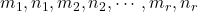be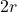integers such that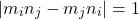for any two integersand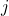satisfying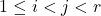. Determine the maximum possible value of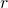.

Solution

INMO 2021, Problem 2

Find all pairs of integers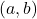so that each of the two cubic polynomials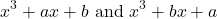has all the roots to be integers.

INMO 2021, Problem 3

Betal marks 2021 points on the plane such that no three are collinear, and draws all possible line segments joining these. He then chooses any 1011 of these line segments, and marks their midpoints. Finally, he chooses a line segment whose midpoint is not marked yet, and challenges Vikram to construct its midpoint using only a straightedge. Can Vikram always complete this challenge?

Note: A straightedge is an infinitely long ruler without markings, which can only be used to draw the line joining any two given distinct points.

INMO 2021, Problem 4

A Magician and a Detective play a game. The Magician lays down cards numbered from 1 to 52 face-down on a table. On each move, the Detective can point to two cards and inquire if the numbers on them are consecutive. The Magician replies truthfully. After a finite number of moves the Detective points to two cards. She wins if the numbers on these two cards are consecutive, and loses otherwise.

Prove that the Detective can guarantee a win if and only if she is allowed to ask at least 50 questions.

INMO 2021, Problem 5

In a convex quadrilateral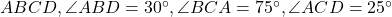and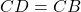. Extend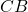to meet the circumcircle of triangleat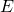. Prove thatSolution

INMO 2021, Problem 6

Letbe the set of all polynomials with real coefficients, and let deg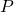denote the degree of a nonzero polynomial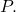Find all functions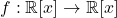satisfying the following conditions:

•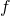maps the zero polynomial to itself,
• for any non-zero polynomial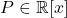,, and
for any two polynomials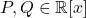, the polynomials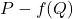and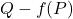have the same set of real roots.

This is a work in progress. Please come back soon for more updates. We are adding problems, solutions and discussions on INMO (Indian National Math Olympiad 2021)

INMO 2021, Problem 1

Supposeis an integer, and letbeintegers such thatfor any two integersandsatisfying. Determine the maximum possible value of.

Solution

INMO 2021, Problem 2

Find all pairs of integersso that each of the two cubic polynomialshas all the roots to be integers.

INMO 2021, Problem 3

Betal marks 2021 points on the plane such that no three are collinear, and draws all possible line segments joining these. He then chooses any 1011 of these line segments, and marks their midpoints. Finally, he chooses a line segment whose midpoint is not marked yet, and challenges Vikram to construct its midpoint using only a straightedge. Can Vikram always complete this challenge?

Note: A straightedge is an infinitely long ruler without markings, which can only be used to draw the line joining any two given distinct points.

INMO 2021, Problem 4

A Magician and a Detective play a game. The Magician lays down cards numbered from 1 to 52 face-down on a table. On each move, the Detective can point to two cards and inquire if the numbers on them are consecutive. The Magician replies truthfully. After a finite number of moves the Detective points to two cards. She wins if the numbers on these two cards are consecutive, and loses otherwise.

Prove that the Detective can guarantee a win if and only if she is allowed to ask at least 50 questions.

INMO 2021, Problem 5

In a convex quadrilateraland. Extendto meet the circumcircle of triangleat. Prove thatSolution

INMO 2021, Problem 6

Letbe the set of all polynomials with real coefficients, and let degdenote the degree of a nonzero polynomialFind all functionssatisfying the following conditions:

•maps the zero polynomial to itself,
• for any non-zero polynomial,, and
for any two polynomials, the polynomialsandhave the same set of real roots.

This site uses Akismet to reduce spam. Learn how your comment data is processed.

### Knowledge Partner